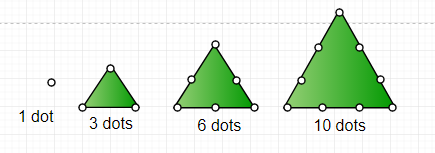GeeksforGeeks App
Open AppBrowser
Continue

# Program to print triangular number series till n

A triangular number or triangle number counts objects arranged in an equilateral triangle, as in the diagram on the right. The n-th triangular number is the number of dots composing a triangle with n dots on a side, and is equal to the sum of the n natural numbers from 1 to n.Examples :

```Input : 5
Output : 1 3 6 10 15

Input : 10
Output : 1 3 6 10 15 21 28 36 45 55

Explanation :
For k = 1 and j = 1 -> print k ( i.e. 1);
increase j by 1 and add into k then print k ( i.e  3 ) update k
increase j by 1 and add into k then print k ( i.e  6 ) update k
increase j by 1 and add into k then print k ( i.e 10 ) update k
increase j by 1 and add into k then print k ( i.e 15 ) update k
increase j by 1 and add into k then print k ( i.e 21 ) update k
.
.
and so on.```

Approach used is very simple. Iterate for loop till the value given n and for each iteration increase j by 1 and add it into k, which will simply print the triangular number series till n.
Below is the program implementing above approach:

## C++

 `// C++ Program to find Triangular Number Series``#include ``using` `namespace` `std;``// Function to find triangular number``void` `triangular_series(``int` `n)``{``    ``int` `i, j = 1, k = 1;` `    ``// For each iteration increase j by 1``    ``// and add it into k``    ``for` `(i = 1; i <= n; i++) {``        ``cout << k << ``" "``;``        ``j = j + 1; ``// Increasing j by 1``        ``k = k + j; ``// Add value of j into k and update k``    ``}``}``// Driven Function``int` `main()``{``    ``int` `n = 5;``    ``triangular_series(n);``    ``return` `0;``}``//this code is contributed by aditya942003patil`

## C

 `// C Program to find Triangular Number Series``#include ` `// Function to find triangular number``void` `triangular_series(``int` `n)``{``    ``int` `i, j = 1, k = 1;` `    ``// For each iteration increase j by 1``    ``// and add it into k``    ``for` `(i = 1; i <= n; i++) {``        ``printf``(``" %d "``, k);``        ``j = j + 1; ``// Increasing j by 1``        ``k = k + j; ``// Add value of j into k and update k``    ``}``}``// Driven Function``int` `main()``{``    ``int` `n = 5;``    ``triangular_series(n);``    ``return` `0;``}`

## Java

 `// Java Program to print triangular number series till n``import` `java.util.*;` `class` `GFG {``    ` `    ``// Function to find triangular number``    ``static` `void` `triangular_series(``int` `n)``    ``{``        ``int` `i, j = ``1``, k = ``1``;``     ` `        ``// For each iteration increase j by 1``        ``// and add it into k``        ``for` `(i = ``1``; i <= n; i++) {` `            ``System.out.printf(``"%d "``, k);``            ``j = j + ``1``; ``// Increasing j by 1``            ``k = k + j; ``// Add value of j into k and update k``        ``}``    ``}``    ` `    ``// Driver function``    ``public` `static` `void` `main(String[] args)``    ``{``            ``int` `n = ``5``;``            ``triangular_series(n);``    ``}``}``        ` `// This code is contributed by Arnav Kr. Mandal.`

## Python3

 `# Python3 code to find Triangular``# Number Series` `# Function to find triangular number``def` `triangular_series( n ):``    ``j ``=` `1``    ``k ``=` `1``    ` `    ``# For each iteration increase j``    ``# by 1 and add it into k``    ``for` `i ``in` `range``(``1``, n ``+` `1``):``        ``print``(k, end ``=` `' '``)``        ``j ``=` `j ``+` `1` `# Increasing j by 1``        ` `        ``# Add value of j into k and update k``        ``k ``=` `k ``+` `j``        ` `# Driven Code``n ``=` `5``triangular_series(n)` `# This code is contributed by "Sharad_Bhardwaj"`

## C#

 `// C# Program to print triangular``// number series till n``using` `System;` `class` `GFG {``    ` `    ``// Function to find triangular number``    ``static` `void` `triangular_series(``int` `n)``    ``{``        ``int` `i, j = 1, k = 1;``    ` `        ``// For each iteration increase j by 1``        ``// and add it into k``        ``for` `(i = 1; i <= n; i++) {` `            ``Console.Write(k +``" "``);``            ``j += 1; ``// Increasing j by 1``            ``k += j; ``// Add value of j into k and update k``        ``}``    ``}``    ` `    ``// Driver Code``    ``public` `static` `void` `Main()``    ``{``            ``int` `n = 5;``            ``triangular_series(n);``    ``}``}``        ` `// This code is contributed by vt_m.`

## PHP

 ``

## Javascript

 ``

Output :

`1 3 6 10 15`

Time complexity : O(n)
Auxiliary Space : O(1), since no extra space has been taken.
Alternate Solution :
The solution is based on the fact that i-th Triangular number is sum of first i natural numbers, i.e., i * (i + 1)/2

## C++

 `// C++ Program to find Triangular Number Series``#include ``using` `namespace` `std;``// Function to find triangular number``void` `triangular_series(``int` `n)``{``    ``for` `(``int` `i = 1; i <= n; i++)``        ``cout << i*(i+1)/2 << ``" "``;``}` `// Driven Function``int` `main()``{``    ``int` `n = 5;``    ``triangular_series(n);``    ``return` `0;``}``//this code is contributed by aditya942003patil`

## C

 `// C Program to find Triangular Number Series``#include ` `// Function to find triangular number``void` `triangular_series(``int` `n)``{``    ``for` `(``int` `i = 1; i <= n; i++)``        ``printf``(``" %d "``, i*(i+1)/2);``}` `// Driven Function``int` `main()``{``    ``int` `n = 5;``    ``triangular_series(n);``    ``return` `0;``}`

## Java

 `//Java program to print triangular number series till n``import` `java.util.*;` `class` `GFG {``    ` `    ``// Function to find triangular number``    ``static` `void` `triangular_series(``int` `n)``    ``{``        ``for` `(``int` `i = ``1``; i <= n; i++)``            ``System.out.printf(``"%d "``;, i*(i+``1``)/``2``);``    ``}``    ` `    ``// Driver function``    ``public` `static` `void` `main(String[] args)``    ``{``            ``int` `n = ``5``;``            ``triangular_series(n);``    ``}``}``        ` `//This code is contributed by Arnav Kr. Mandal.`

## Python3

 `# Python3 code to find Triangular``# Number Series`` ` `def` `triangular_series(n):`` ` `     ``for` `i ``in` `range``(``1``, n ``+` `1``):``         ``print``( i``*``(i``+``1``)``/``/``2``,end``=``' '``)`` ` `# Driver code``n ``=` `5``triangular_series(n)``# This code is contributed by ihritik`

## C#

 `// C# program to print triangular``// number series till n``using` `System;` `class` `GFG {``    ` `    ``// Function to find triangular number``    ``static` `void` `triangular_series(``int` `n)``    ``{``        ``for` `(``int` `i = 1; i <= n; i++)``            ``Console.Write(i * (i + 1) / 2 + ``" "``);``    ``}``    ` `    ``// Driver Code``    ``public` `static` `void` `Main()``    ``{``            ``int` `n = 5;``            ``triangular_series(n);``    ``}``}``        ` `// This code is contributed by vt_m.`

## PHP

 ``

## Javascript

 ``

Output :

`1 3 6 10 15`

Time complexity : O(n)
Auxiliary Space : O(1) , since no extra space has been taken.

My Personal Notes arrow_drop_up